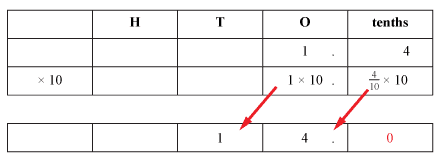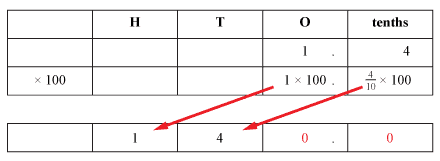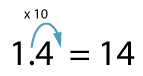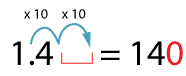#### Multiplying decimal numbers by 10, 100 or 1000

Multiplying decimal numbers by 10, 100 or 1000 follows the same pattern as for multiplying whole numbers by 10, 100 or 1000.

1.4 × 10 =Detailed description

1.4 × 10 = 14

1.4 × 100 =Detailed description

So 1.4 × 100 = 140

Again, the pattern continues:

1.4 × 10 = 14
1.4 × 100 = 140
1.4 × 1000 = 1400
1.4 × 10 000 = 14 000
and so on.

You may have noticed a shortcut:

When you multiply a decimal number by ten, the decimal point moves one place to the right.

For 1.4 × 10:When you multiply a decimal by a hundred, the decimal point moves two places to the right. Multiplying by 100 is the same as multiplying by 10 two times, so we make two jumps to the right.

For 1.4 × 100:To multiply a number such as 1.4 × 100, the decimal point moves two places to the right and we fill the ones place with a zero to say that there are 'no ones'.